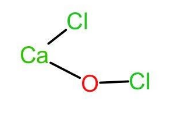QuestionAnswers

# In bleaching powder $(CaO{Cl}_{2})$, oxidation number of the two chlorine atom are:(A) 0, +1(B) 0, -1(C) -1, +1(D) -1, -2

Hint: In bleaching powder, $(CaO{Cl}_{2})$ the two chlorine atoms are attached to two different atoms and due to this they have different oxidation states. The oxidation number of an atom is defined as the charge that atom would have possessed if the compound was composed of ions.

Complete answer step by step: The oxidation number or oxidation state is the total no. of electrons that an atom either loses or gains in order to form chemical bonds with another atom. If the oxidation number is positive then that means the atom loses electrons and if it is negative it means that it gains the electrons.
Let us now look at bleaching powder. The chemical name of bleaching powder is calcium hypochlorite or calcium oxychloride and thus has a chemical formula as $CaO{Cl}_{2}$. It acts as a bleaching agent in aqueous solutions only. In bleaching powder, $(CaO{Cl}_{2})$, the two chlorine atoms are attached to two different atoms. One of the chlorine atoms is attached to calcium and the other chlorine atom is attached to oxygen. Thus, the chemical formula can also be written as $Ca(OCl)Cl$. The structure of the same is given below:Let the oxidation state of Cl attached to calcium be x and oxidation state of Cl in $^{-}OCl$ be y.
We know that Ca is bivalent, therefore,
x = -1 and
2x + y = -1
Substituting the value of x, we get,
2(-1) + y = -1
$\implies$ y = +1
Therefore, the oxidation states of the two chlorine atoms in bleaching powder is -1 and +1.

Hence, option (c) is the correct answer.

Note: It is very important to note that oxidation numbers are always found out for individual atoms and not for groups of atoms. Therefore, in the above case, +1 is not the oxidation number of the group $^{-}OCl$ but of chlorine in $^{-}OCl$. The oxygen in $^{-}OCl$ has oxidation no. of -2.